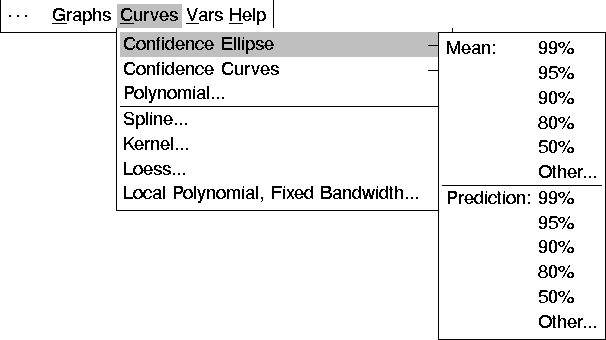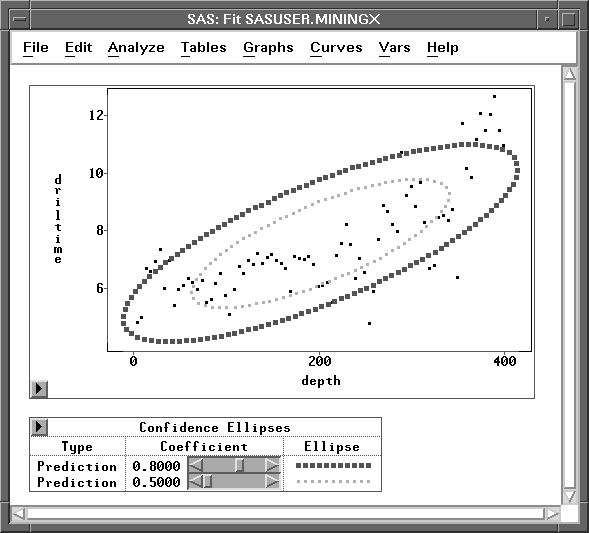Fit Analyses

## Parametric Curves: Confidence Ellipses

SAS/INSIGHT software provides two types of confidence ellipses for each pair of X and Y variables assuming a bivariate normal distribution. One is a confidence ellipse for the population mean, and the other is a confidence ellipse for prediction.

Let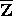and S be the sample mean and the unbiased estimate of the covariance matrix of a random sample of size n from a bivariate normal distribution with mean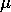and covariance matrix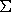.

The variable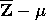is distributed as a bivariate normal variate with mean 0 and covariance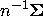,and it is independent of S. The confidence ellipse foris based on Hotelling's T2 statistic: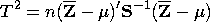A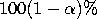confidence ellipse foris defined by the equation
where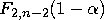is the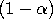critical value of an F variate with degrees of freedom 2 and n-2.

A confidence ellipse for prediction is a confidence region for predicting a new observation in the population. It also approximates a region containing a specified percentage of the population.

Consider Z as a bivariate random variable for a new observation. The variable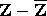is distributed as a bivariate normal variate with mean 0 and covariance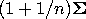,and it is independent of S.

Aconfidence ellipse for prediction is then given by the equation
The family of ellipses generated by different F critical values has a common center (the sample mean) and common major and minor axes.

The ellipses graphically indicate the correlation between two variables. When the variable axes are standardized (by dividing the variables by their respective standard deviations), the ratio of the two axis lengths (in Euclidean distances) reflects the magnitude of the correlation between the two variables. A ratio of 1 between the major and minor axes corresponds to a circular confidence contour and indicates that the variables are uncorrelated. A larger value of the ratio indicates a larger positive or negative correlation between the variables.

You can choose the level of the confidence region from the Confidence Ellipse menus, as illustrated by Figure 39.34.